Function Repository Resource:

# TaylorPolynomial

Compute the Taylor polynomial of a given order of a function of one or several variables

Contributed by: Dennis M Schneider
 ResourceFunction["TaylorPolynomial"][f,{x,x0,n}] computes the Taylor polynomial of the function f of a single variable about the point x0 of degree n. ResourceFunction["TaylorPolynomial"][f,{x,y,…},{x0,y0,…},n] computes the Taylor polynomial of the function f of several variables about the point (x0,y0,…) of degree n.

## Details and Options

With some functions it is necessary to use limits to compute the terms of the series. For these functions, set the option "UseLimit" to True.

## Examples

### Basic Examples (4)

A simple example:

 In:=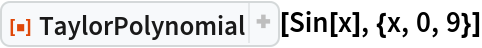Out=It returns the same result as:

 In:=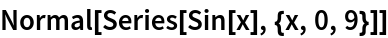Out=An example of a Taylor polynomial of a function of three variables:

 In:=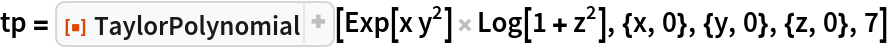Out=Compare with Mathematica's Series command. The two commands treat the expansion differently; TaylorPolynomial expands to a total degree of each term, while Series expands in each variable separately:

 In:=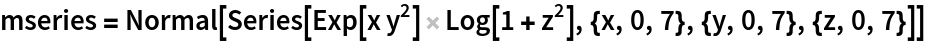Out=Here is the difference between the two commands:

 In:=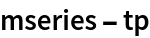Out=The Taylor polynomial of a function of two variables:

 In:=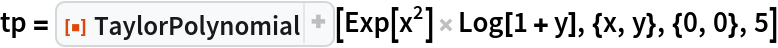Out=Plot the function together with its fifth degree Taylor polynomial:

 In:=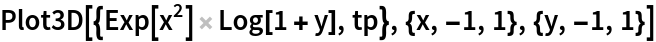Out=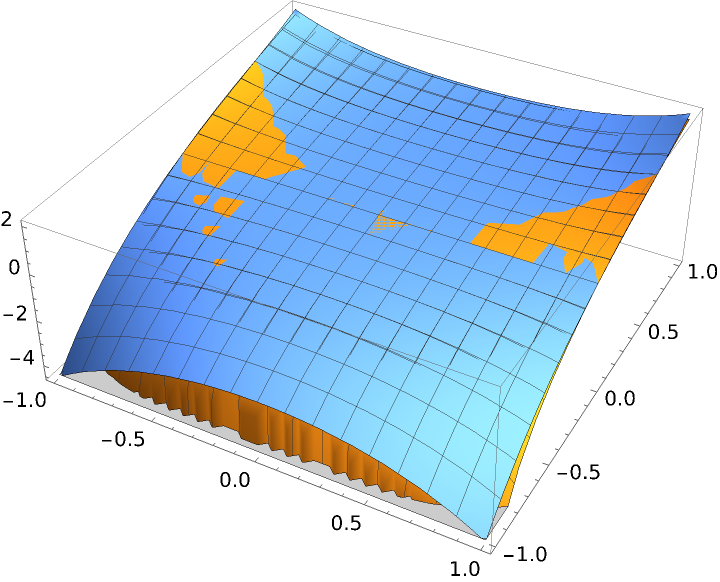The Taylor polynomial of a function with a removable singularity at the origin:

 In:=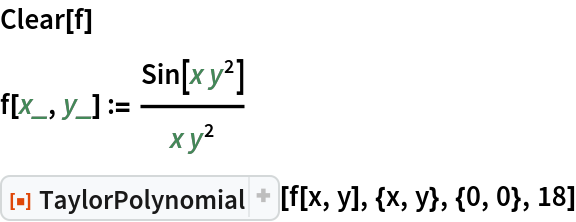Out=This can be obtained by making a simple substitution in a univariate function:

 In:=Out=### Scope (3)

The Taylor polynomial of degree 2 for a symbolic function centered at (a,b):

 In:=Out=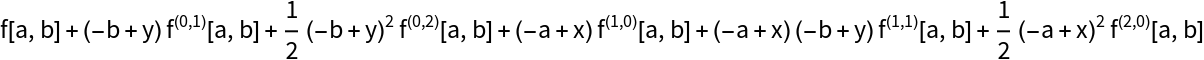An infinitely differentiable function of one variable that is not the zero function but all of whose Taylor polynomials are 0:

 In:=Out=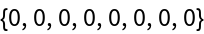Without using limits:

 In:=Out=An infinitely differentiable function of two variables that is not the zero function but all of whose Taylor polynomials are 0:

 In:=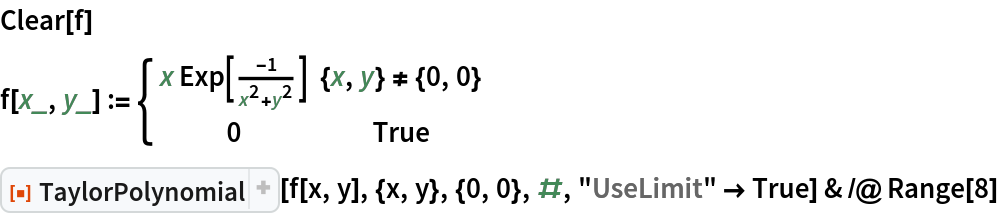Out=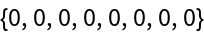Without using limits:

 In:=Out=## Publisher

Dennis M Schneider

## Version History

• 1.0.0 – 15 August 2022